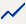1
 2 Select the variable or variables that you want to move to the new axis.
 3 Repeat the process to plot additional variables against the new axis.
The CrimeData.jmp sample data table contains data on various types of crime for the 50 US states yearly, from 1973 to 2010. The Total column sums the number of incidents, and the Total Rate column gives a population-adjusted rate. You want to show these two variables on a single chart.
 1 Select Help > Sample Data Library and open CrimeData.jmp.
 2 Select Graph > Graph Builder.
 3 Select Total Rate and Total and drag them to the Y zone.
 4 Select Year and drag it to the X zone.
Figure 2.14 Total Rate and Total Merged
The Total Rate values are all between 0 and 1, and so are barely visible. Calculate yearly means and then use a second Y axis to make these values visible.
 5 In the options panel for Points, select Mean next to Summary Statistic.
 6 Click the Line element.
 7 Right-click on the variable names in the Y zone and select Move Right > Total Rate.
Figure 2.15 Second Y Axis Added
An axis for Total Rate is added on the right, and the axes rescale to show both sets of values. You can now see relationships between the two measures. For example, both measures began to decrease in 1991.
 8 (Optional) Click Done.

Help created on 7/12/2018